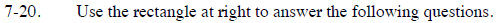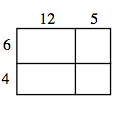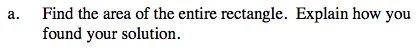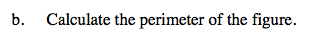### Home > CC3 > Chapter 7 > Lesson 7.1.2 > Problem7-20

7-20.
1.  a. 6x − y = 4 3x + y = 5 1. (0, –4) b. x = y + 4 2x + 3y = −12 2. (3, 7) c. 5x − 2y = 1 y = 2x + 1 3. (1, 2)
2.  12 5 6 4

Use the rectangle at right to answer the following questions. Homework Help ✎

1. Find the area of the entire rectangle. Explain how you found your solution.

2. Calculate the perimeter of the figure.The area of a figure is the same as the sum of the area of its parts.

72 + 30 + 48 + 20The perimeter of a figure is the sum of its sides.

54 units